Latest Banking jobs   »

# Quantitative Aptitude Quiz For IBPS RRB PO/Clerk Mains 2022- 15th September

Directions (1-5): Solve the given quadratic equations and mark the correct option based on your answer—
(a) x > y
(b) x ≥ y
(c) x < y
(d) x ≤ y
(e) x = y or no relation can be established between x and y.

Q1. I. 3x² – 10x + 8 = 0
II. 5y² – 22y + 24 = 0

Q2. I. 4x² + 39x + 90 = 0
II. 2y² – 3√3 y-15 =0

Q3. I. 5x² – 36 = 12²
II. y² + 17y + 72 = 0

Q4. I. 1.5x² – 10x + 16 =0
II. y² = 6y

Q5. I. 2x² – 21x + 55 = 0
II. 5y² – 46y + 105 = 0

Directions (6-10): In each question two equations numbered (I) and (II) are given. Student should solve both the equations and mark appropriate answer.

Q6. I. x³ = –512
II. 2y² + 31y + 120 = 0
(a) x ≥ y
(b) no relation can be established between x and y.
(c) x > y
(d) x < y
(e) x ≤ y

Q7. I. x²=∛15625
II. (y – 3)²= 5-y
(a) x ≤ y
(b) x > y
(c) x ≥ y
(d) x < y
(e) no relation can be established between x and y.

Q8.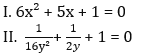(a) no relation can be established between x and y
(b) x ≥ y
(c) x ≤ y
(d) x > y
(e) x < y

Q9. I. (x – 6)² = 49
II. y² + 8y = 84
(a) x ≥ y
(b) x < y
(c) no relation can be established between x and y
(d) x > y
(e) x ≤ y

Q10.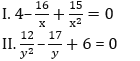(a) x ≤ y
(b) x > y
(c) x ≥ y
(d) no relation can be established between x and y
(e) x < y

Directions (11-15): Solve the given quadratic equations and mark the correct option based on your answer—
(a) x < y
(b) x ≤ y
(c) x = y or no relation can be established between x and y.
(d) x > y
(e) x ≥ y

Q11. (i) x²-25x+156=0
(ii) y²-21y+108=0

Q12. (i) 3x + 5y = 18
(ii)7x + 8y = 42

Q13. (i)2x² + 15x + 27 = 0
(ii)3y² + 25y – 18 = 0

Q14. (i)6x² + 29x + 35 =0
(ii)20y² + 27y + 9 =0

Q15. (i)x² – 7x – 30 = 0
(ii)y² + 15y + 50 = 0

Solutions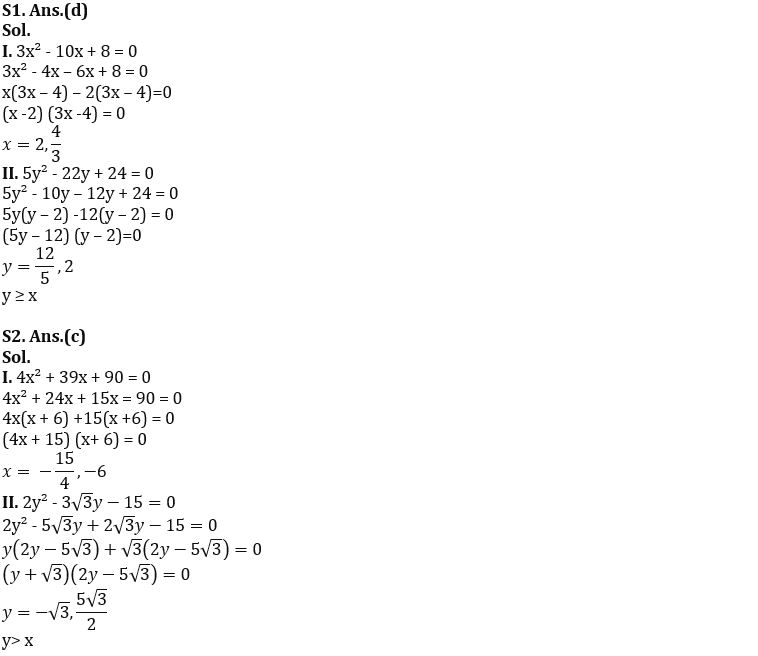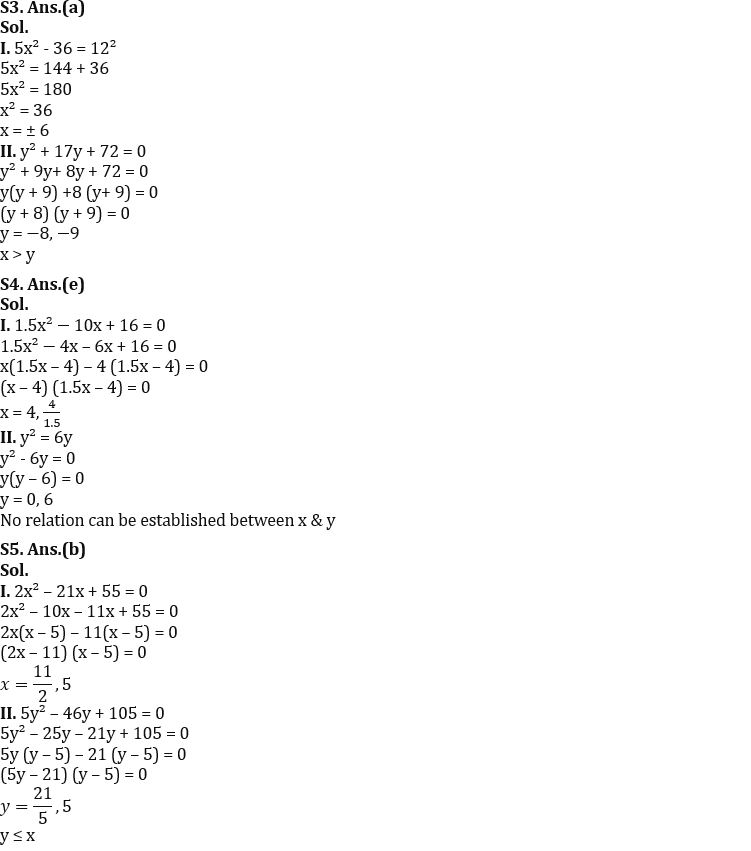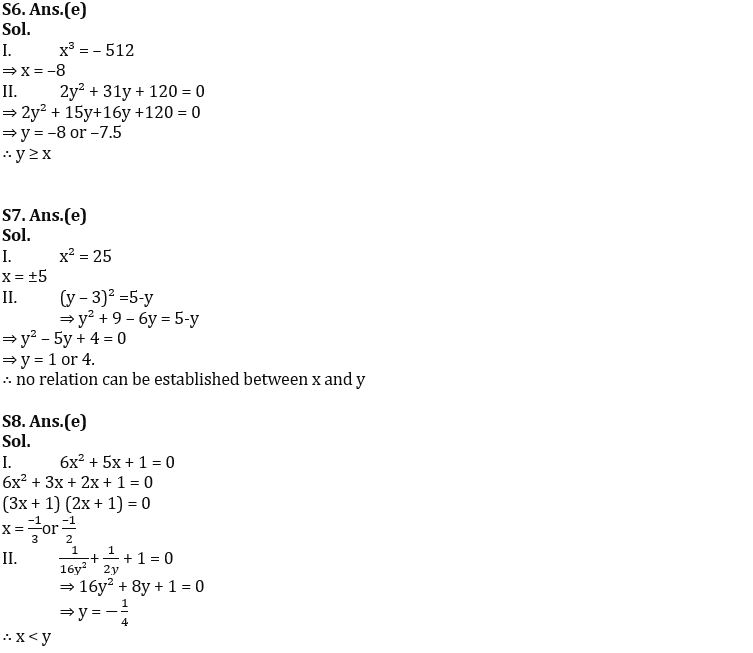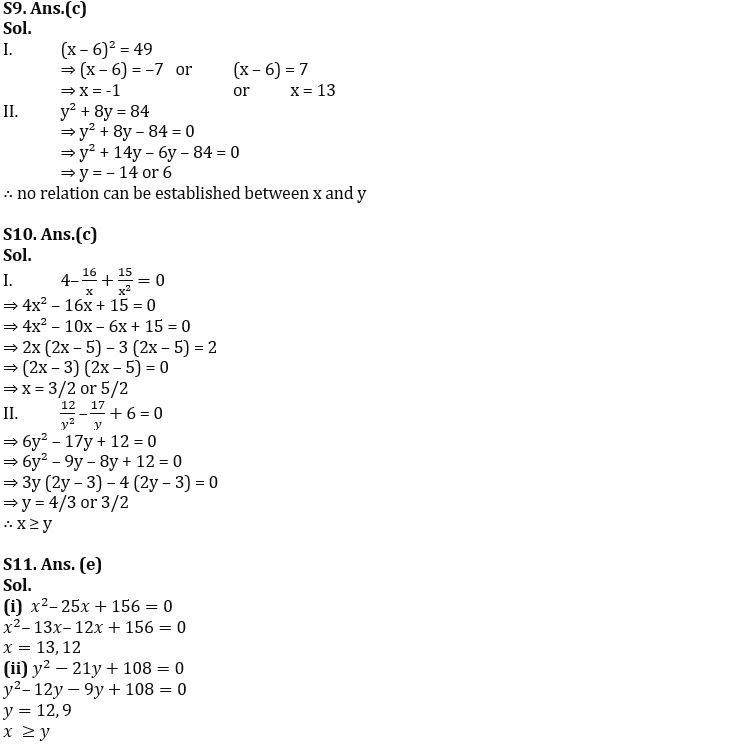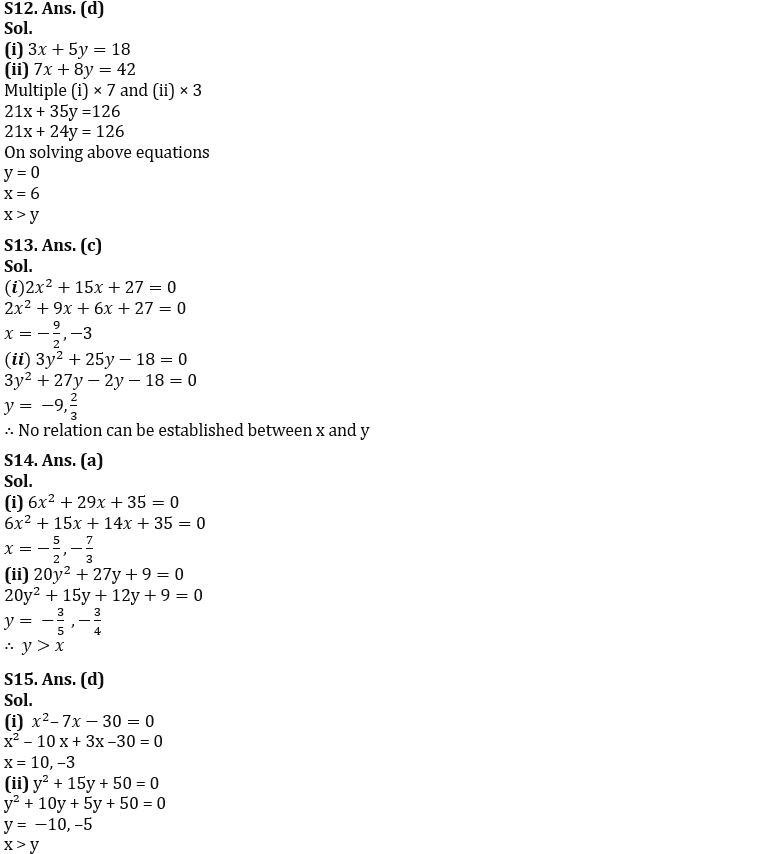#### Congratulations!Download Hindu Review of October 2021: Free PDF